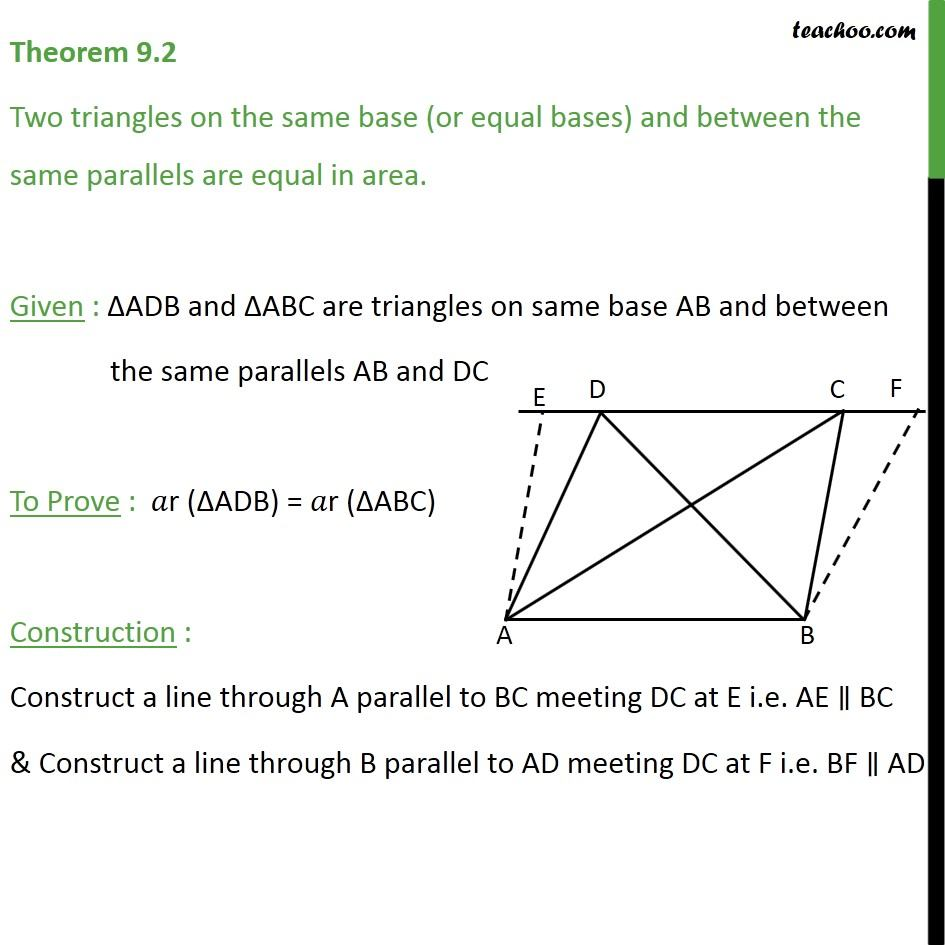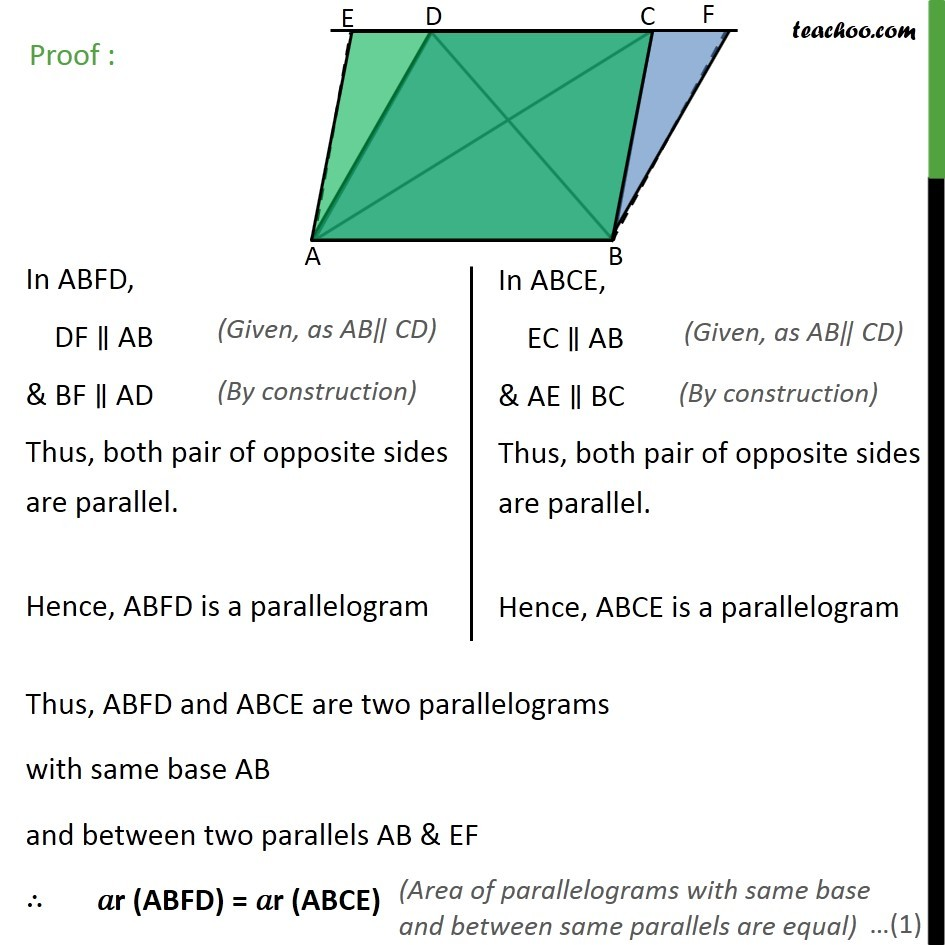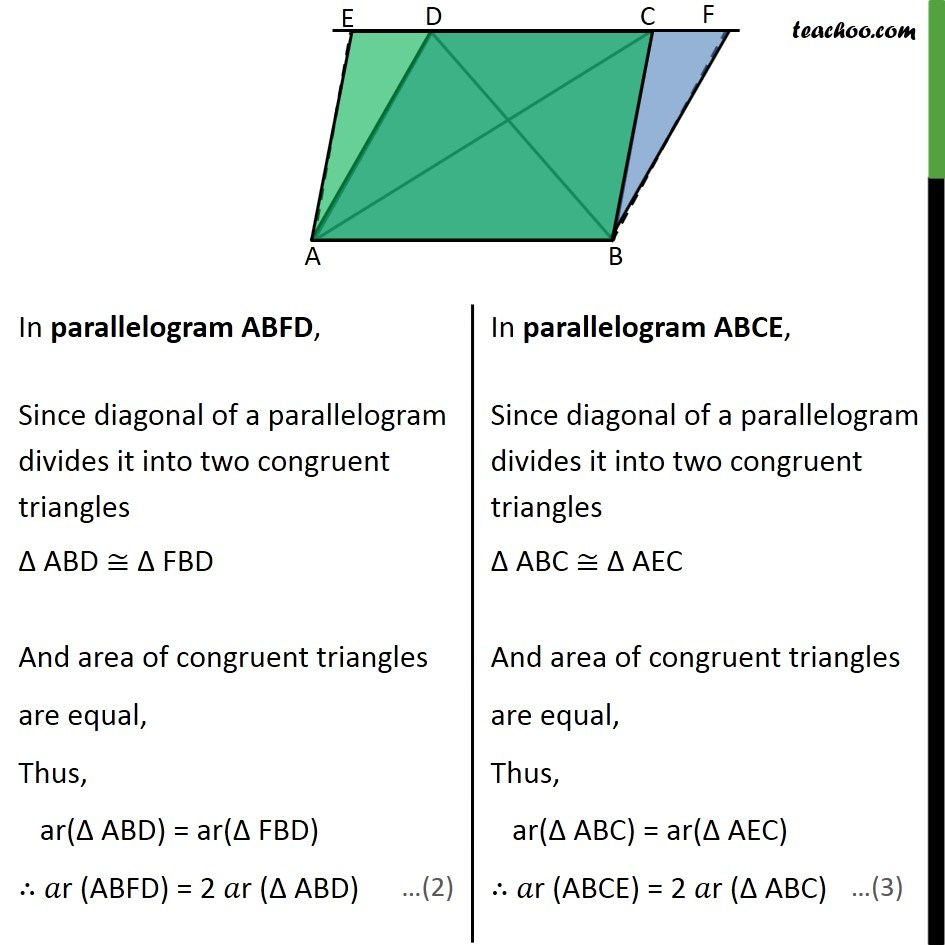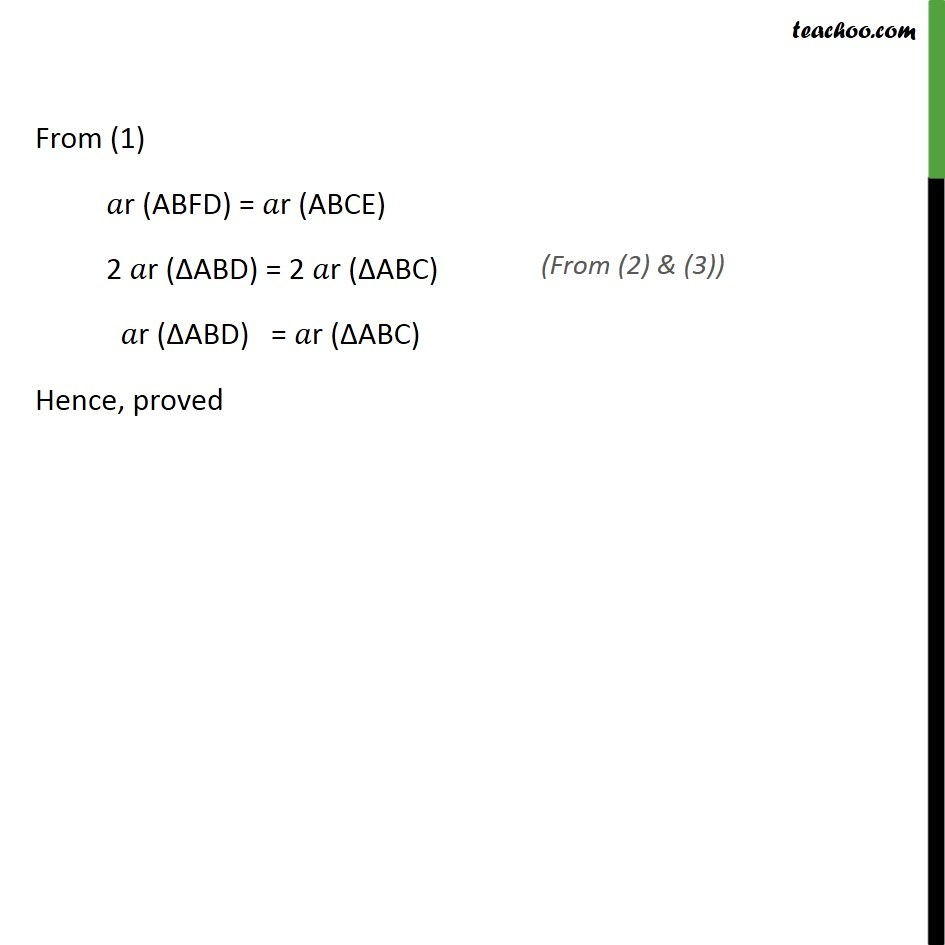Subscribe to our Youtube Channel - https://you.tube/teachoo

1. Chapter 9 Class 9 Areas of Parallelograms and Triangles
2. Concept wise
3. Triangles with same base & same parallel lines

Transcript

Theorem 9.2 (Method 1) Two triangles on the same base (or equal bases) and between the same parallels are equal in area. Given : ∆ADB and ∆ABC are triangles on same base AB and between the same parallels AB and DC To Prove : 𝑎r (∆ADB) = 𝑎r (∆ABC) Proof: We know that Area of triangle = 1/2×𝐵𝑎𝑠𝑒×𝐻𝑒𝑖𝑔ℎ𝑡 Here AB is base We draw DE ⊥ AB So, DE is the height of ∆ ABD ∴ Area ∆ ABD = 1/2×𝐵𝑎𝑠𝑒×𝐻𝑒𝑖𝑔ℎ𝑡 = 1/2×𝐴𝐵×𝐷𝐸 Here AB, is base We draw CF ⊥ AB So, CF is the height of ∆ ABC ∴ Area ∆ ABC = 1/2×𝐵𝑎𝑠𝑒×𝐻𝑒𝑖𝑔ℎ𝑡 = 1/2×𝐴𝐵×𝐶𝐹 DE & CF are perpendicular between the same parallel line ∴ DE = CF So, from (1) Area ∆ ABD = 1/2×𝐴𝐵×𝐷𝐸 = 1/2×𝐴𝐵×𝐶𝐹 = Area ∆ ABC ∴ Area ∆ ABD = Area of ∆ ABC Hence proved. Theorem 9.2 Two triangles on the same base (or equal bases) and between the same parallels are equal in area. Given : ∆ADB and ∆ABC are triangles on same base AB and between the same parallels AB and DC To Prove : 𝑎r (∆ADB) = 𝑎r (∆ABC) Construction : Construct a line through A parallel to be meeting DC at E i.e. AE ∥ BC & Construct a line through B parallel to AD meeting DC at F i.e. BF ∥ AD Proof : From (1) 𝑎r (ABFD) = 𝑎r (ABCE) 2 𝑎r (∆ABD) = 2 𝑎r (∆ABC) 𝑎r (∆ABD) = 𝑎r (∆ABC) Hence, proved

Triangles with same base & same parallel lines

About the AuthorDavneet Singh
Davneet Singh is a graduate from Indian Institute of Technology, Kanpur. He has been teaching from the past 9 years. He provides courses for Maths and Science at Teachoo.